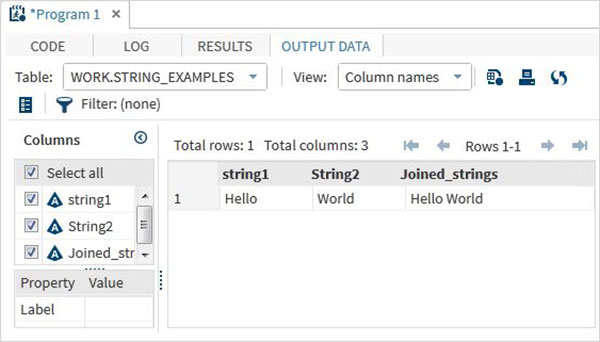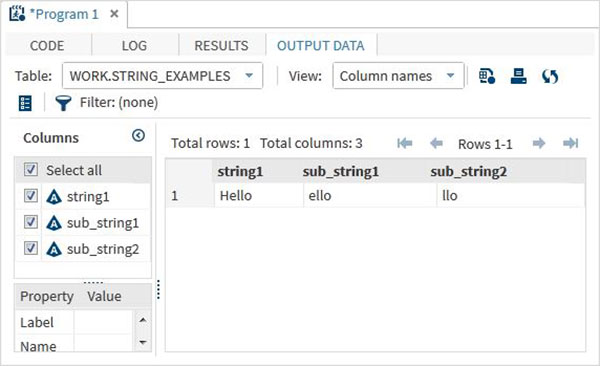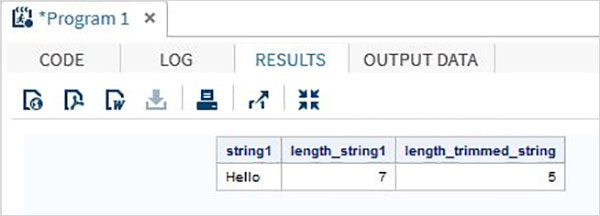# SAS - Strings

Strings in SAS are the values which are enclosed with in a pair of single quotes. Also the string variables are declared by adding a space and \$ sign at the end of the variable declaration. SAS has many powerful functions to analyze and manipulate strings.

## Declaring String Variables

We can declare the string variables and their values as shown below. In the code below we declare two character variables of lengths 6 and 5. The LENGTH keyword is used for declaring variables without creating multiple observations.

```data string_examples;
LENGTH string1 \$ 6 String2 \$ 5;
/*String variables of length 6 and 5 */
String1 = 'Hello';
String2 = 'World';
Joined_strings =  String1 ||String2 ;
run;
proc print data = string_examples noobs;
run;
```

On running the above code we get the output which shows the variable names and their values.## String Functions

Below are the examples of some SAS functions which are used frequently.

## SUBSTRN

This function extracts a substring using the start and end positions. In case of no end position is mentioned it extracts all the characters till end of the string.

### Syntax

```SUBSTRN('stringval',p1,p2)
```

Following is the description of the parameters used −

• stringval is the value of the string variable.
• p1 is the start position of extraction.
• p2 is the final position of extraction.

### Example

```data string_examples;
LENGTH string1 \$ 6 ;
String1 = 'Hello';
sub_string1 = substrn(String1,2,4) ;
/*Extract from position 2 to 4 */
sub_string2 = substrn(String1,3) ;
/*Extract from position 3 onwards */
run;
proc print data = string_examples noobs;
run;
```

On running the above code we get the output which shows the result of substrn function.### TRIMN

This function removes the trailing space form a string.

### Syntax

```TRIMN('stringval')
```

Following is the description of the parameters used −

• stringval is the value of the string variable.
```data string_examples;
LENGTH string1 \$ 7  ;
String1='Hello  ';
length_string1 = lengthc(String1);
length_trimmed_string = lengthc(TRIMN(String1));
run;
proc print data = string_examples noobs;
run;
```

On running the above code we get the output which shows the result of TRIMN function.